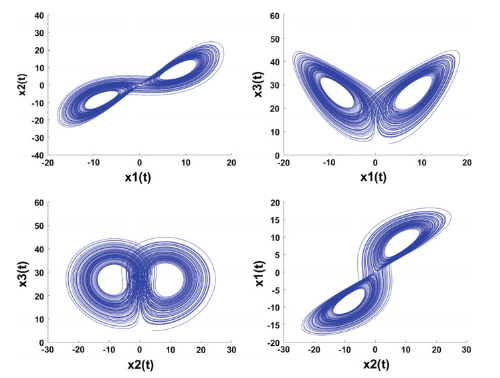Journal

# On new fractional inverse matrix projective synchronization schemes

By
Ouannas A.
Azar A.T.
Ziar T.
Vaidyanathan S.

In this study, the problem of inverse matrix projective synchronization (IMPS) between different dimensional fractional order chaotic systems is investigated. Based on fractional order Lyapunov approach and stability theory of fractional order linear systems, new complex schemes are proposed to achieve inverse matrix projective synchronization (IMPS) between n-dimension and m-dimension fractional order chaotic systems. To validate the theoretical results and to verify the effectiveness of the proposed schemes, numerical applications and computer simulations are used. © Springer International Publishing AG 2017.